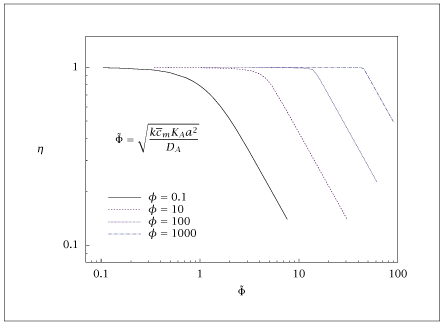## Figure 7.13:

### Effectiveness factor versus an inappropriate Thiele modulus in a slab; Hougen-Watson kinetics.## Code for Figure 7.13

### main.m

```
1
2
3
4
5
6
7
8
9
10
11
12
13
14
15
16
17
18
19
20
21
22
23
24
25
26
27
28
29
30
31
32
33
34
35
36
37
38
39
40
41
42
43
44
45
46
47
48
49
50
51
52
53
54
55
56
57
58
59
60
61
62
63
64
65
66
67
68
69
70
71
72
73
74
75
76
77
78
79
80
81
82
83
84
85
86
87
88
89
90
91
92
93
94
95
96
97
98
99
100
101
102
103
104
105
106
107
108
109
110
111
112
113
114
115
116
117
118
119
120
121
122
123
124
125
126
127
128
129
130
131
132
133
134
135
136
137
138
139
140
141
142
143
144
145
146
147
148
149
150
151
152
153
154% Copyright (C) 2001, James B. Rawlings and John G. Ekerdt
%
% This program is free software; you can redistribute it and/or
% modify it under the terms of the GNU General Public License as
% published by the Free Software Foundation; either version 2, or (at
% your option) any later version.
%
% This program is distributed in the hope that it will be useful, but
% WITHOUT ANY WARRANTY; without even the implied warranty of
% MERCHANTABILITY or FITNESS FOR A PARTICULAR PURPOSE.  See the GNU
% General Public License for more details.
%
% You should have received a copy of the GNU General Public License
% along with this program; see the file COPYING.  If not, write to
% the Free Software Foundation, 59 Temple Place - Suite 330, Boston,
% MA 02111-1307, USA.

%
% Try to solve for the dimensionless pellet profile using
% a Langmuir Hinshelwood mechanism.
%
% d^2 c/ d r^2 -Phi^2 c / (1 + phi c) = 0
% c(1)      = 1
% dc/dr (0) = 0
%
% goal is to not use a shooting method to avoid numerical problems
% try it as a pure ODE
%
% jbr, 6/12/01
%

% first effort: consider dimensional problem
%
% x1 = c_A
% x2 = dc_A/dr
%
% dx2/dr = p1 p2 c_A / (1 + p2 c_A)
% dx1/dr = x2
%
% x1(0) = c0
% x2(0) = 0
%
% solve this IVP to r=r1 and x1=c1
%
% p1 = k cm/De
% p2 = KA
% Phi^2 = p1*p2*r1^2
% phi = p2*c1
%
% problem up to here is both Phi and phi will change with r1,c1
% make p2 change with time as an ODE as well, to keep phi=constant
% dp2/dr = -p2*x2/x1
% p2(0)  = phi/x1(0)
%
% OK, don't think the above idea of changing p2 is solving the right problem.
% Just march the ODE to desired phi, change p2 and solve ODE again
% to same phi.  The two values of Phi should be different, however,
% and that allows a family of Phi at constant phi. Drawback is that
% we are solving the ODE a lot.
%
% Revised 8/22/2018

p1 = 1;
p2 = 1;
c0 = 0.1;
p.p1 = p1; p.p2 = p2;

x0 = [c0; 0; 0; 0];
npts = 200;
rstop = 10;
rsteps = linspace(0.001,rstop,npts)';

phivec = [0.1 10 100 1000];
nphi = length(phivec);
results = [];

for j = 1:nphi
% March ODE solver out to phi stopping condition for a range of p2
%
% next pairs go together for smooth log log plot of etavec vs. Phivec

phi = phivec(j);
p.phi = phi;
p2vec = [1e-40 1e-10 .1 1 10 20 30 40 50 55 60 65 70 75 80 90 95 99 99.5];

if (phi >= 100)
p2vec(1) = 1e-20;
end

np2 = length(p2vec);
c0 = 1e-2*phi;
Phivec = zeros(np2,1);
etavec = zeros(np2,1);

for i = 1:np2
p2 = p2vec(i);
p.p2 = p2;
rstop = max(100,100*sqrt(1/p2));
rsteps = [0; linspace(0.001,rstop,npts)'];
x0 = [c0; 0; 0; 0];

opts = odeset('Events', @(t, x) g(t,x,p), ...
'AbsTol', sqrt(eps), 'RelTol', sqrt(eps));
[rout,x] = ode15s (@(t,x) hougensrt(t,x,p), rsteps, x0, opts);

if ( length(rout) == npts + 1)
fprintf ('hey, did not reach requested phi value, increase rstop\n');
end

nout = length(rout);
cbar = x(:,1)/x(nout,1);
rbar = rout/rout(nout);
%  plot (rbar,cbar);
Phivec(i) = sqrt(p1*p2)*rout(nout);
etavec(i) = (1 + phi)*x(nout,2)*rout(nout)/x(nout,1)/Phivec(i)^2;
%
% check, should be zero if solved the dim'less  model
% hmm, apparently cannot trust the last xdot.  Could make a call
% to hougensrt to correct this last  one if this were important
% check passed, 6/13/01
%
% ode15s doesn't return xdot at all the solution times, so would
% need to compute it here to check.
%
% check = rout(nout)^2/x(nout,1)*xdot(:,2) - ...
% Phivec(i)^2*cbar./(1+phi*cbar);
%    fprintf ('norm of error in solution: %g\n', max(abs(check(1:nout-1))));
end

Phiprimevec = phi/(1 + phi) / sqrt( 2*(phi - log(1+phi)) ) * Phivec;
results = [results Phivec Phiprimevec etavec];
end

save -ascii etahougwat.dat results;

if (~ strcmp (getenv ('OMIT_PLOTS'), 'true')) % PLOTTING
subplot (2, 1, 1);
loglog (results(:,1), results(:,3), ...
results(:,4), results(:,6), ...
results(:,7), results(:,9), ...
results(:,10), results(:,12));
axis ([0.07, 100, .08, 1.5]);
% TITLE etahougwat

subplot (2, 1, 2);
loglog (results(:,2), results(:,3), ...
results(:,5), results(:,6), ...
results(:,8), results(:,9), ...
results(:,11), results(:,12));
axis ([0.07, 10, .08, 1.5]);
% TITLE etahougwatasy
end % PLOTTING

```

### hougensrt.m

```
1
2
3
4
5
6
7
8
9function xdot = hougensrt(t, x, p)
ca    = x(1);
s1    = x(3);
s2    = x(4);
p.p1*p.p2*ca/(1 + p.p2*ca);
s2;
p.p1*ca/(1 + p.p2*ca)^2 + p.p1*p.p2*s1/(1 + p.p2*ca)^2];

```

### g.m

```
1
2
3
4
5function [stp, isterminal, direction] = g(t, x, p)
phipellet = p.p2*x(1);
stp = phipellet - p.phi;
isterminal = 1;
direction = 0;

```